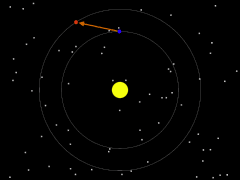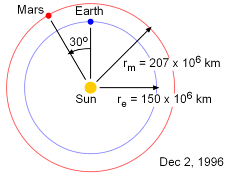Ch 2. Vectors Multimedia Engineering Statics Scalars & Vectors 2-DVectors 3-DVectors DotProducts
 Chapter 1. Basics 2. Vectors 3. Forces 4. Moments 5. Rigid Bodies 6. Structures 7. Centroids/Inertia 8. Internal Loads 9. Friction 10. Work & Energy Appendix Basic Math Units Sections Search eBooks Dynamics Fluids Math Mechanics Statics Thermodynamics Author(s): Kurt Gramoll ©Kurt GramollSTATICS - CASE STUDY IntroductionProblem Graphic NASA is planning an unmanned mission to Mars. Mission planners need the precise position of the planet relative to Earth on the launch date in order to determine the proper velocity for the spacecraft. If the distance or angle is incorrect, the spacecraft could be lost. What is known: The radius of Earth's orbit re is 150 x 106 km. The radius of Mars's orbit rm is 207 x 106 km. The angle α between Earth and Mars relative to the Sun is 30°. Assume both Earth's and Mars' orbits around the Sun is circular. QuestionProblem Diagram What is the position of Mars relative to Earth on the launch day? Approach Draw a diagram and label the key points and known position vectors. Determine the relationship between the unknown position vector and those that are given. Using geometry, solve for the length and angle of the unknown vector.

Practice Homework and Test problems now available in the 'Eng Statics' mobile app
Includes over 500 problems with complete detailed solutions.
Available now at the Google Play Store and Apple App Store.